• 如图，若给出每个文本框的坐标，而我们想将其剪裁下来，可以使用如下方法。 import numpy as np import cv2 def shot(img, dt_boxes,image_file):#通过dt_boxes中获得的四个坐标点,裁剪出图像 dt_boxes = dt_...
如图，若给出每个文本框的坐标，而我们想将其剪裁下来，可以使用如下方法。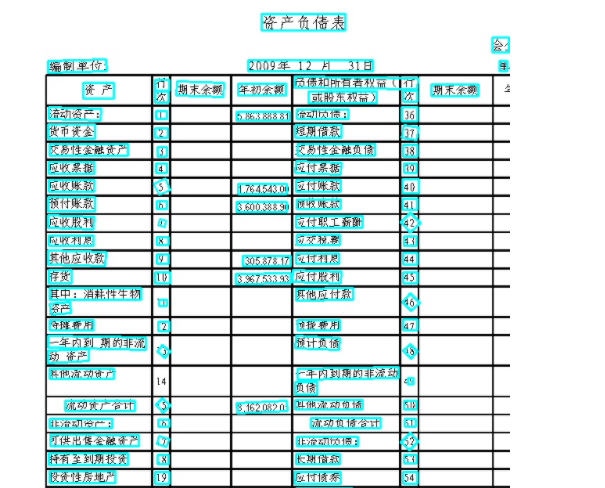import numpy as np
import cv2

def shot(img, dt_boxes,image_file):#通过dt_boxes中获得的四个坐标点,裁剪出图像
dt_boxes = dt_boxes.tolist() #将np转换为列表
boxes_len = len(dt_boxes)
for num in range(boxes_len):
box = dt_boxes[num]
tl = box
tr = box
br = box
bl = box
crop = img[int(tr):int(bl), int(tl):int(br) ]
try:
cv2.imwrite("./mydata/b/"+image_file.split("/")[-1][:-4]+"_"+str(num) + ".jpg", crop)
except:
continue
num = num + 1




展开全文字符串 opencv leetcode 列表
• python matplotlib坐标轴设置的方法 在使用matplotlib模块时画坐标图时，往往需要对坐标轴设置很多参数，这些参数包括横纵坐标轴范围、坐标轴刻度大小、坐标轴名称等 在matplotlib中包含了很多函数，用来对这些参数...

python matplotlib坐标轴设置的方法

在使用matplotlib模块时画坐标图时，往往需要对坐标轴设置很多参数，这些参数包括横纵坐标轴范围、坐标轴刻度大小、坐标轴名称等
在matplotlib中包含了很多函数，用来对这些参数进行设置。
我们可以对坐标轴进行设置，设置坐标轴的范围，设置坐标轴上的文字描述等。
基本用法
例如：
import numpy as np
import pandas as pd
import matplotlib.pyplot as plt
生成x轴上的数据:从-3到3，总共有50个点
x = np.linspace(-1, 1, 50)
定义一个线性方程
y1 = 2 * x + 1
定义一个二次方程
y2 = x ** 2
设置x轴的取值范围为：-1到2
plt.xlim(-1, 2)
设置y轴的取值范围为：-1到3
plt.ylim(-1, 3)
设置x轴的文本，用于描述x轴代表的是什么
plt.xlabel(“I am x”)
设置y轴的文本，用于描述y轴代表的是什么
plt.ylabel(“I am y”)
plt.plot(x, y2)
绘制红色的线宽为1虚线的线条
plt.plot(x, y1, color=‘red’, linewidth=1.0, linestyle=’–’)
显示图表
plt.show()
输出图为：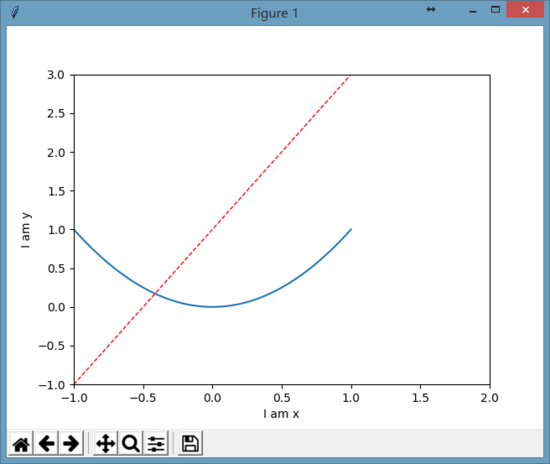修改坐标轴的刻度
想要修改x轴的刻度，从-1到2，总共5点：
new_ticks = np.linspace(-1, 2, 5)
plt.xticks(new_ticks)
只要把上述代码放在画图之前，最后画出来的图为：
这样x轴上的刻度就被修改成了从-1到2共5个点。
把刻度用文字来表示
设置y刻度：用文字来显示刻度
plt.yticks([-2, -1.8, -1, 1.22, 3],
[‘really bad’, ‘bad’, ‘normal’, ‘good’, ‘really good’])
上面代码的意思就是把y刻度中的数值和文字进行了映射：
-2 => ‘really bad’
-1.8 => ‘bad’
-1 => ‘normal’
1.22 => ‘good’
3 => ‘really good’
显示图为：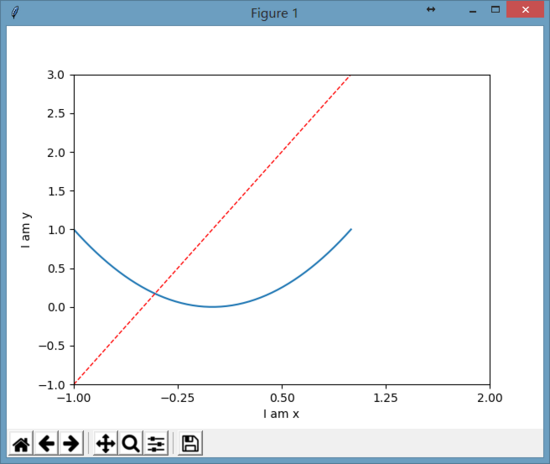修改坐标轴刻度字体
为了能够使刻度上的字体显示得好看些，可以使用：
plt.yticks([-2, -1.8, -1, 1.22, 3],
[r’$really\ bad$’, r’$bad\alpha$’, ‘normal’, r’$good$’, ‘really good’])
上面用正则文本方式来显示字体，同时还用\alpha来转义显示数学中的alpha字母，图像显示如下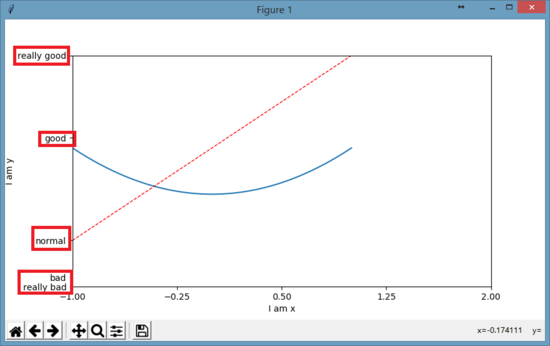以上就是本文的全部内容，希望对大家的学习有所帮助，也希望大家多多支持脚本之家。


展开全文• 我想要写一个自动点击屏幕的脚本，要点击某段文字，怎么获取这个文字坐标啊？</p>
• 今天小编就为大家分享一篇python matplotlib绘图,修改坐标轴刻度为文字的实例，具有很好的参考价值，希望对大家有所帮助。一起跟随小编过来看看吧
•展开全文• 工作中偶尔需要做客流分析，用pyplot 库绘图。一般情况下， x 轴刻度...#_ = plt.xticks(scale_ls,index_ls) ## 可以设置坐标字 plt.title('Average customer flows Number by Weekdays')
工作中偶尔需要做客流分析，用pyplot 库绘图。一般情况下， x 轴刻度默认显示为数字。例如：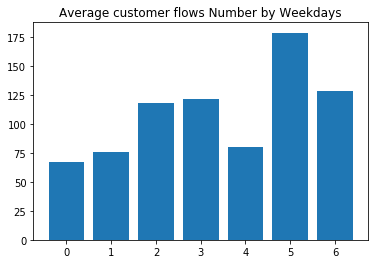我希望x 轴刻度显示为星期日期。
查询pyplot 文档， 发现了 xtick() 函数可以修改刻度。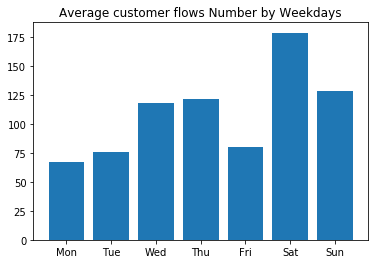代码如下：
import matplotlib.pyplot as plt
import numpy as np
#val_ls = [np.random.randint(100) + i*20 for i in range(7)]
scale_ls = range(7)
index_ls = ['Mon','Tue','Wed','Thu','Fri','Sat','Sun']
plt.bar(scale_ls, val_ls)
#_ = plt.xticks(scale_ls,index_ls)  ## 可以设置坐标字
plt.title('Average customer flows Number by Weekdays')


展开全文pyplot matplotlib Ipython numpy
• 运行环境：python3.6 第三方库：PIL (安装命令：pip install pillow) 该项目主要目的：将图中中文替换成英文 ...图片中部分文字坐标 字典数据：（图片左上角是原点（0，0）,x轴是横轴，y轴是纵轴。） dic...
• python可视化作堆积条形图，以及将坐标值改为文字、改变文字颜色，旋转文字。 具体代码如下： import numpy as np import pylab as pl pl.rcParams['font.sans-serif']=['SimHei'] n=12 xn=["January","February",...可视化 数据可视化 html
• x轴范围是 150000-300000, 直接用plt.plot，图像横坐标过于长， 想要将横坐标替换成 160K, 200K 形式 查了很多方法 但都没有很好解决，但实际上一行代码就可以了 plt.xticks(range(160000,320000,20000), labels=[...matplotlib
• 本文将介绍如何将直方图坐标值改为文字 具体代码如下： import numpy as np import pylab as pl pl.rcParams['font.sans-serif']=['SimHei'] val_ls = [np.random.randint(100) + i*20 for i in range(5)] scale_ls ...数据可视化 eclipse
• 在图上画出注释 ... arrowprops=dict(facecolor='r', frac=0.2, headwidth=30, width=20)) #注释的文字,xy是箭头的坐标，xytext注释的起始坐标 plt.show() 在图中加上文字，并更改相关参数 x = np.ar
• Py之Matplotlib：python包之Matplotlib库图表绘制经验总结(中英文字体修改、横坐标文字进行横/纵向显示、控制坐标轴范围等)之详细攻略 目录 1、Matplotlib库图表绘制包所涉及中文、英文各种字体格式修改集合...
• # 绘制文本 font = ImageFont.truetype("consola.ttf", 40, encoding="unic" ) # 设置字体 ...#坐标参数：第一个是x，第二是y # -*- coding: UTF-8 -*- import numpy as np...
• Python批量识别图片指定区域文字内容简介所需Python模块Python代码 简介 对于一张图片，需求识别指定区域的内容 截取原始图上的指定图片当做模板 根据模板相似度去再原始图片上识别准确坐标 根据坐标剪切出指定位置...图片识别
• 使用python编写的从图像中取点的程序。本程序设定是取得11行39列的文字坐标，如有其它需求可在本基础上改。通过鼠标左键单击获取文字位置，左键双击完成整个一行的取点。鼠标右击完成整个取点过程。
• 效果图1polor标准功能： 源码如下： import pandas as pd import numpy as np import matplotlib....mpl.rcParams['font.sans-serif'] = ['FangSong'] # 指定仿宋中文字体防止文字乱码 r = 2 * np.random.rand(10
• python画图，等间距坐标距离表示不等间距数据值 在做图常遇到数据差距较大，但按实际距离作图时，效果并不好，不能均匀的分布在图上，希望将坐标刻度换成非实际间距，采用将真实坐标换成文字标签的笨方法。 例如：画...数据可视化 数据分析...

# python文字坐标python 订阅